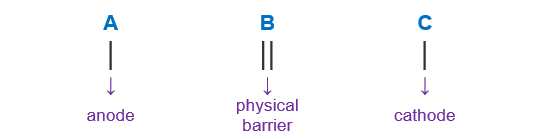# Problem: Consider the following half-reactions and their standard reduction potentials then give the standard line (cell) notation for a voltaic cell built on these half reactions.Mn2+ (aq) + 2 e- ⇌ Mn(s)                   E° = -1.18 VCr3+ (aq) + 3 e-⇌ Cr(s)                      E° = -0.41 Va. Mn (s) | Mn2+(aq, 1.0 M) || Cr3+(aq, 1.0 M) | Cr(s)b. Mn2+(aq, 1.0 M) | Mn (s) || Cr3+(aq, 1.0 M) | Cr(s)c. Mn2+(aq, 1.0 M) | Mn (s) || Cr3+(aq, 1.0 M)d. Cr(s) l Cr3+(aq, 1.0 M) || Mn (s) | Mn2+(aq, 1.0 M)e. Cr3+(aq, 1.0 M) l Cr(s) ll Mn2+(aq, 1.0 M) | Mn (s)

###### FREE Expert Solution

We’re being asked to determine the cell notation for the redox reaction shown below:

Mn2+ (aq) + 2 e- ⇌ Mn(s)                   E° = -1.18 V

Cr3+ (aq) + 3 e-⇌ Cr(s)                      E° = -0.41 V

When writing a cell notation, we use the following format – “as easy as ABCTo determine the cell notation of the reaction, we will use the following steps:
Step 1: Determine the anode and the cathode.
Step 2Write the cell notation of the reaction.

91% (368 ratings)###### Problem Details

Consider the following half-reactions and their standard reduction potentials then give the standard line (cell) notation for a voltaic cell built on these half reactions.

Mn2+ (aq) + 2 e- ⇌ Mn(s)                   E° = -1.18 V

Cr3+ (aq) + 3 e-⇌ Cr(s)                      E° = -0.41 V

a. Mn (s) | Mn2+(aq, 1.0 M) || Cr3+(aq, 1.0 M) | Cr(s)

b. Mn2+(aq, 1.0 M) | Mn (s) || Cr3+(aq, 1.0 M) | Cr(s)

c. Mn2+(aq, 1.0 M) | Mn (s) || Cr3+(aq, 1.0 M)

d. Cr(s) l Cr3+(aq, 1.0 M) || Mn (s) | Mn2+(aq, 1.0 M)

e. Cr3+(aq, 1.0 M) l Cr(s) ll Mn2+(aq, 1.0 M) | Mn (s)

Frequently Asked Questions

What scientific concept do you need to know in order to solve this problem?

Our tutors have indicated that to solve this problem you will need to apply the Cell Notation concept. If you need more Cell Notation practice, you can also practice Cell Notation practice problems.

What is the difficulty of this problem?

Our tutors rated the difficulty ofConsider the following half-reactions and their standard red...as medium difficulty.

What professor is this problem relevant for?

Based on our data, we think this problem is relevant for Professor Zaitsev's class at UH.